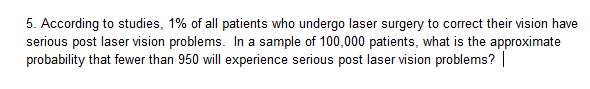# 5. According to studies, 1% of all patients who undergo laser surgery to correct their vision haveserious post laser vision problems. In a sample of 100,000 patients, what is the approximateprobability that fewer than 950 will experience serious post laser vision problems?

Question
12 viewshelp_outlineImage Transcriptionclose5. According to studies, 1% of all patients who undergo laser surgery to correct their vision have serious post laser vision problems. In a sample of 100,000 patients, what is the approximate probability that fewer than 950 will experience serious post laser vision problems? fullscreen
check_circle

Step 1

Introduction to binomial distribution:

Consider a random experiment involving n independent trials, such that the outcome of each trial can be classified as either a “success” or a “failure”. The numerical value “1” is assigned to each success and “0” is assigned to each failure.

Moreover, the probability of getting a success in each trial, p, remains a constant for all the n trials. Denote the probability of failure as q. As success and failure are mutually exclusive, q = 1 – p.

Let the random variable X denote the number of successes obtained from the n trials. Thus, X can take any of the values 0,1,2,…,n.

Then, the probability distribution of X is a Binomial distribution with parameters (n, p) and the probability mass function (pmf) of X, that is, of a Binomial random variable, is given as:

Step 2

Normal approximation to binomial distribution:

It is given that the size of the sample taken from the population of patients is n = 100,000. It is known that the patients will be independent of each other, imp-lying independence among each other. As a result, the 100,000 patients may be considered as 100,000 independent trials.

Consider the event of containing a wine as a “success”. It is said that 1% of patients who undergo laser surgery to correct their vision have serious post laser vision problems. That is, Thus, the probability that a patient who undergo laser surgery to correct their vision have serious post laser vision problems, that is, the probability of success in each trial is p = 0.01. Then q = 1 – 0.01 = 0.99.

Consider x as the number of patients who undergo laser surgery to correct their vision have serious post laser vision problems. Then, x has a Binomial distribution with parameters (n = 100,000, p = 0.01).

The mean and variance for the binomial variate is E(x) = n * p and V(x) = n *p *(1 – p).

Here, the sample size n is large.

The mean and variance of the variable x is E(x) = n * p = 100,000*0.01 = 1,000 and V(x) = n *p *(1 – p) = 100,000*0.01*0.99 = 990.

If x follows binomial distribution with parameters np and npq, the binomial variate tends to normal as follows:

Step 3

Find the probability that fewer than 950 patients experience post laser vision problems:

Here, the requirement is number of patients who undergo laser surgery to correct their vision have serious post laser vision problems should be le...

### Want to see the full answer?

See Solution

#### Want to see this answer and more?

Solutions are written by subject experts who are available 24/7. Questions are typically answered within 1 hour.*

See Solution
*Response times may vary by subject and question.
Tagged in

### Other